# ICSE Class 10 Physics Question Paper Solution 2016

ICSE Class 10 Physics Question Paper Solution 2016 is provided here for making students exam easier and simpler. The solutions contain the answers to each question described in detail along with step marking. The solution pdf will provide a depth understanding of how much to write for a particular answer during the exam and how the marks are awarded to students based on the step marking. This information will help students to plan a proper exam strategy and also prepare them effectively for the paper. The solutions created by the subject experts are helpful for board exam preparation.

The ICSE Class 10 Physics 2016 exam was started at 11 am and students were allotted 2 hours of time duration to finish the paper. They can download the ICSE Class 10 Physics Question Paper Solution 2016 PDF from the link below and refer to it anytime while studying.

Students can have a look at the ICSE Class 10 Physics Question Paper Solution 2016 below: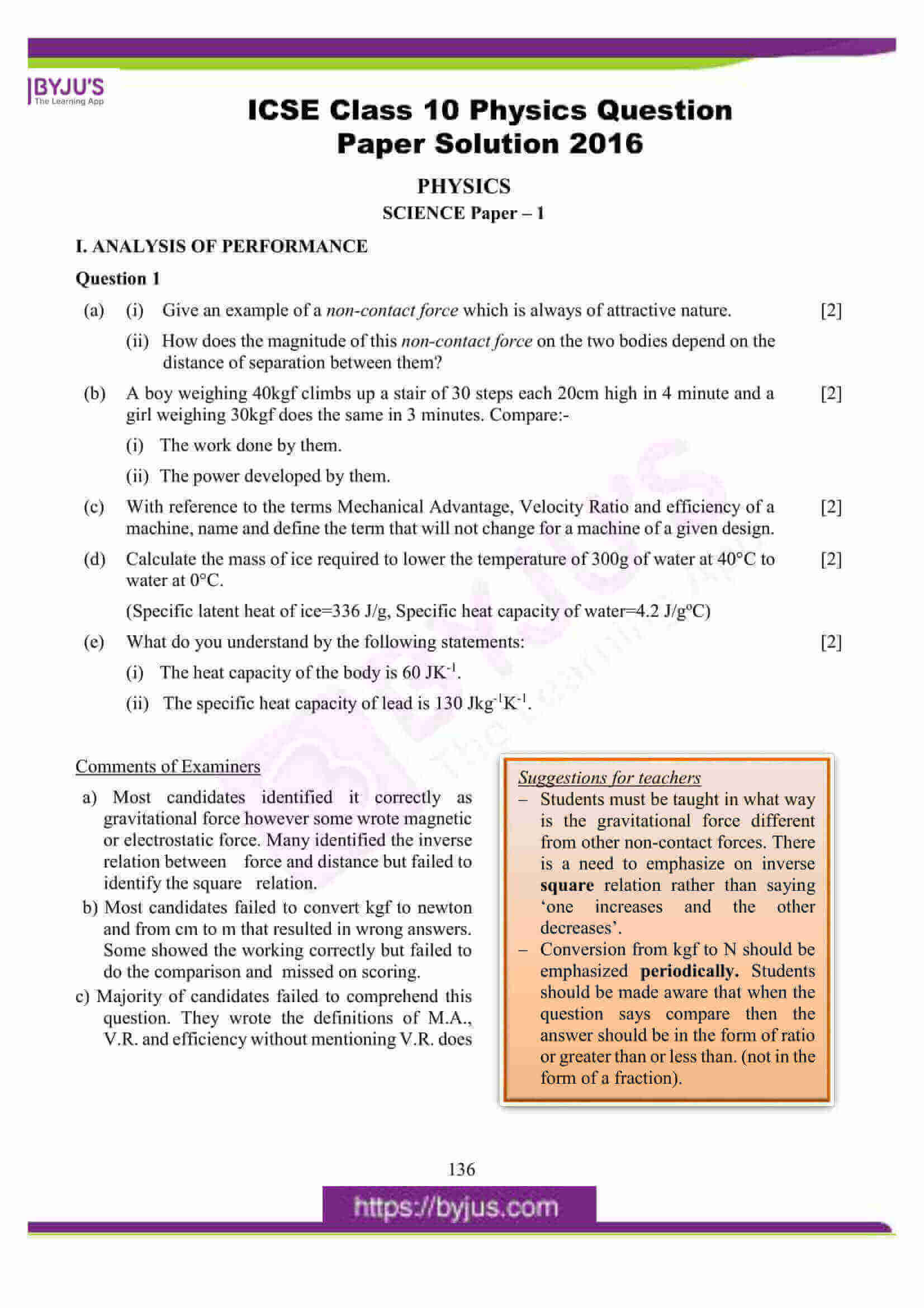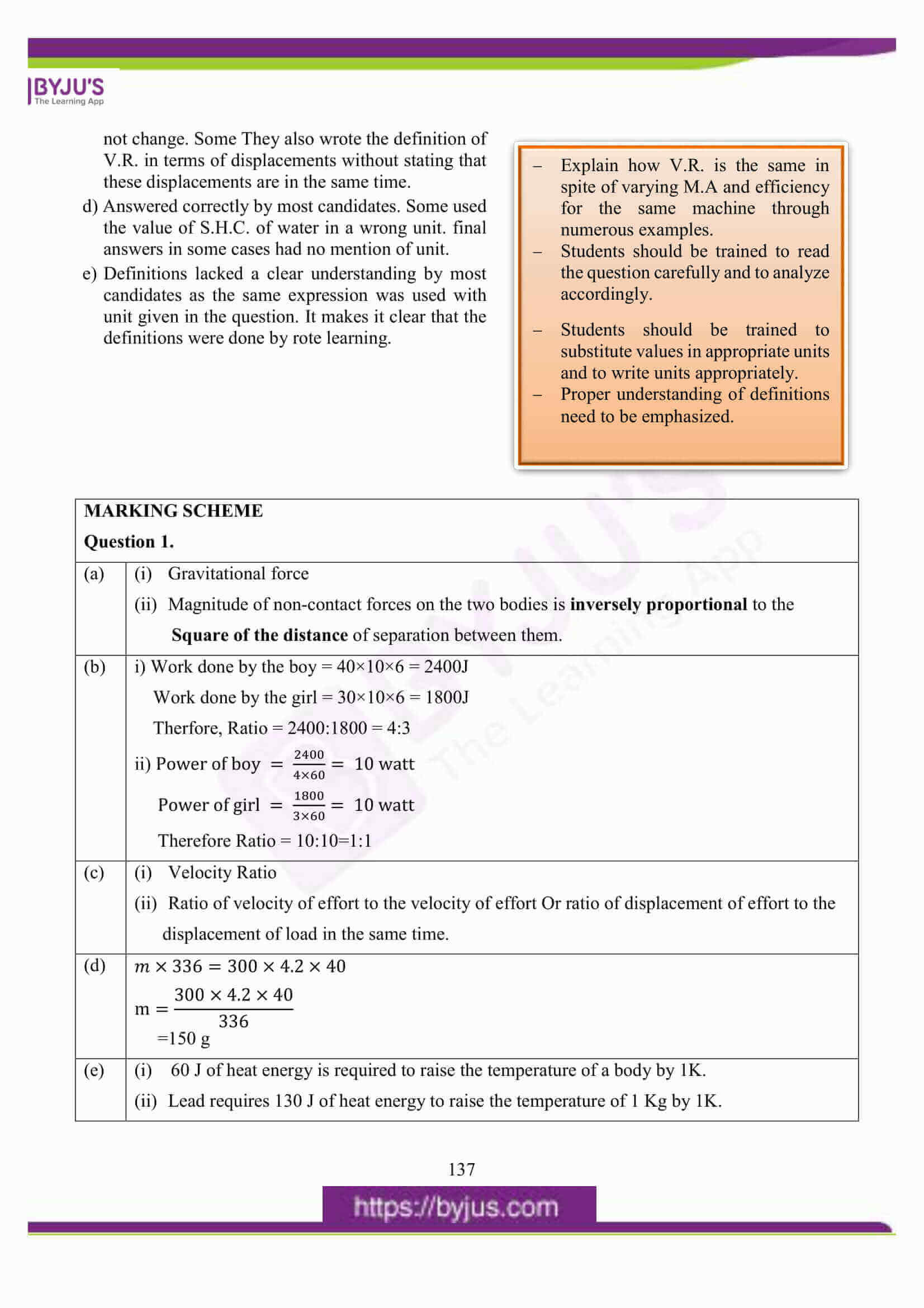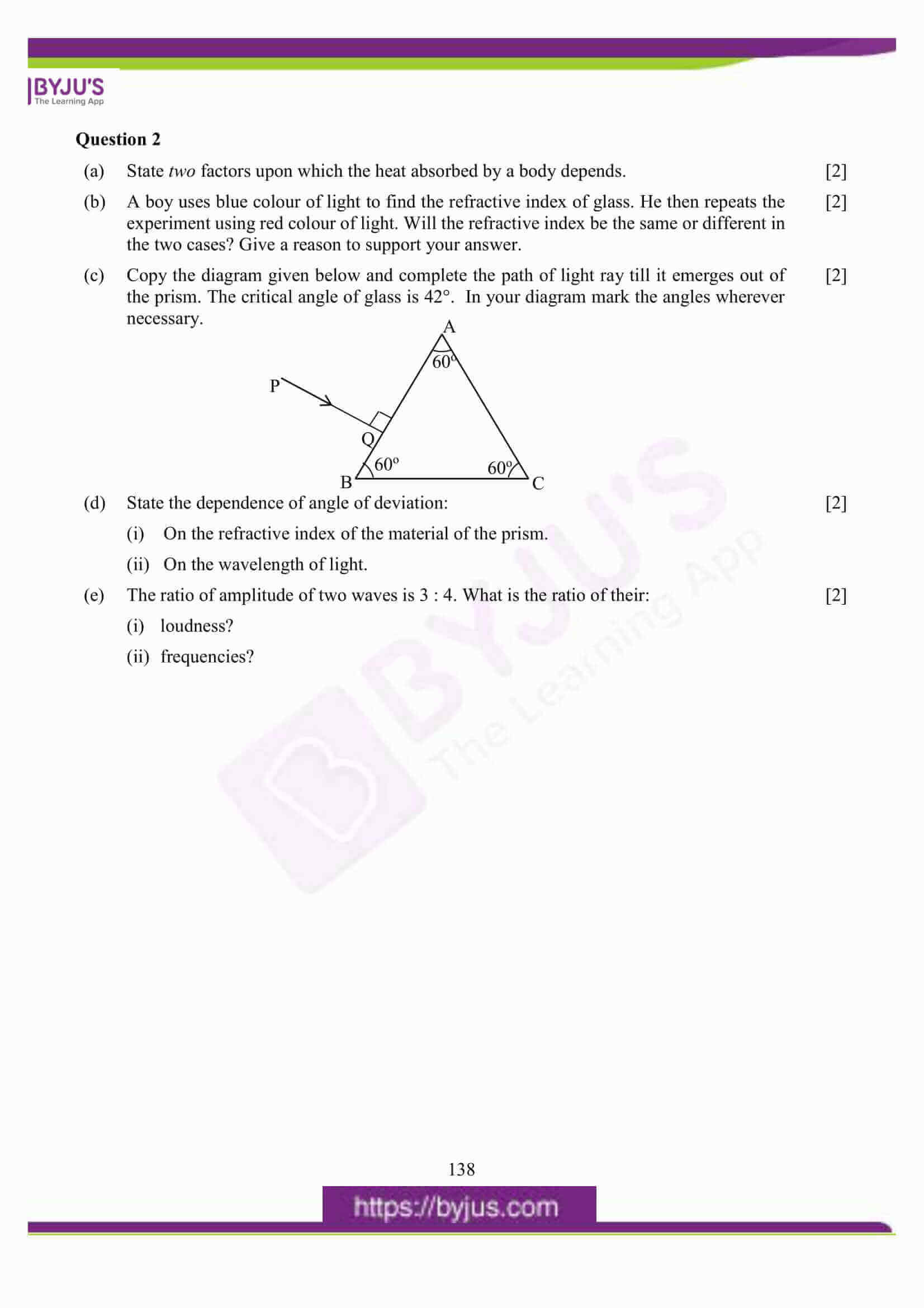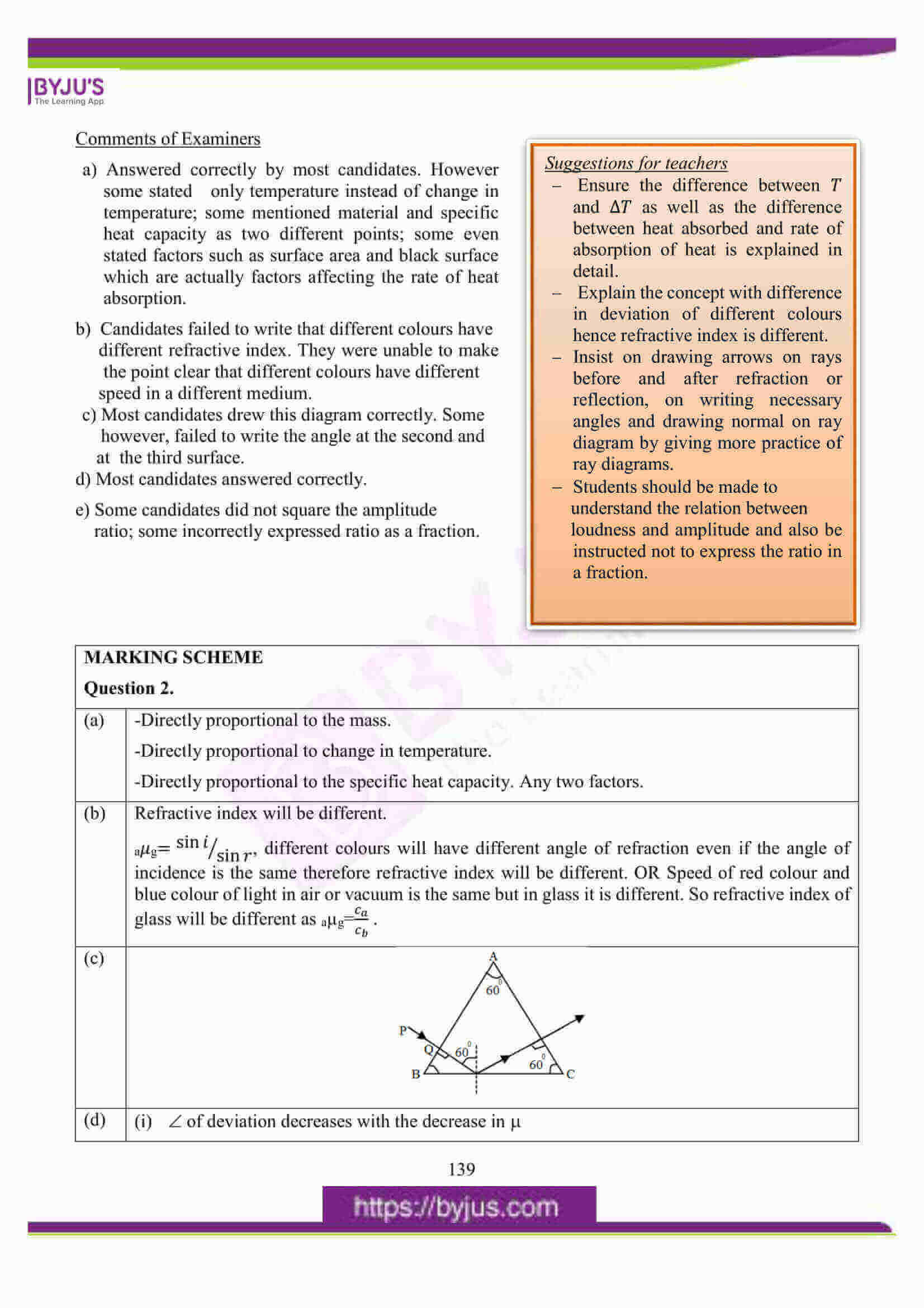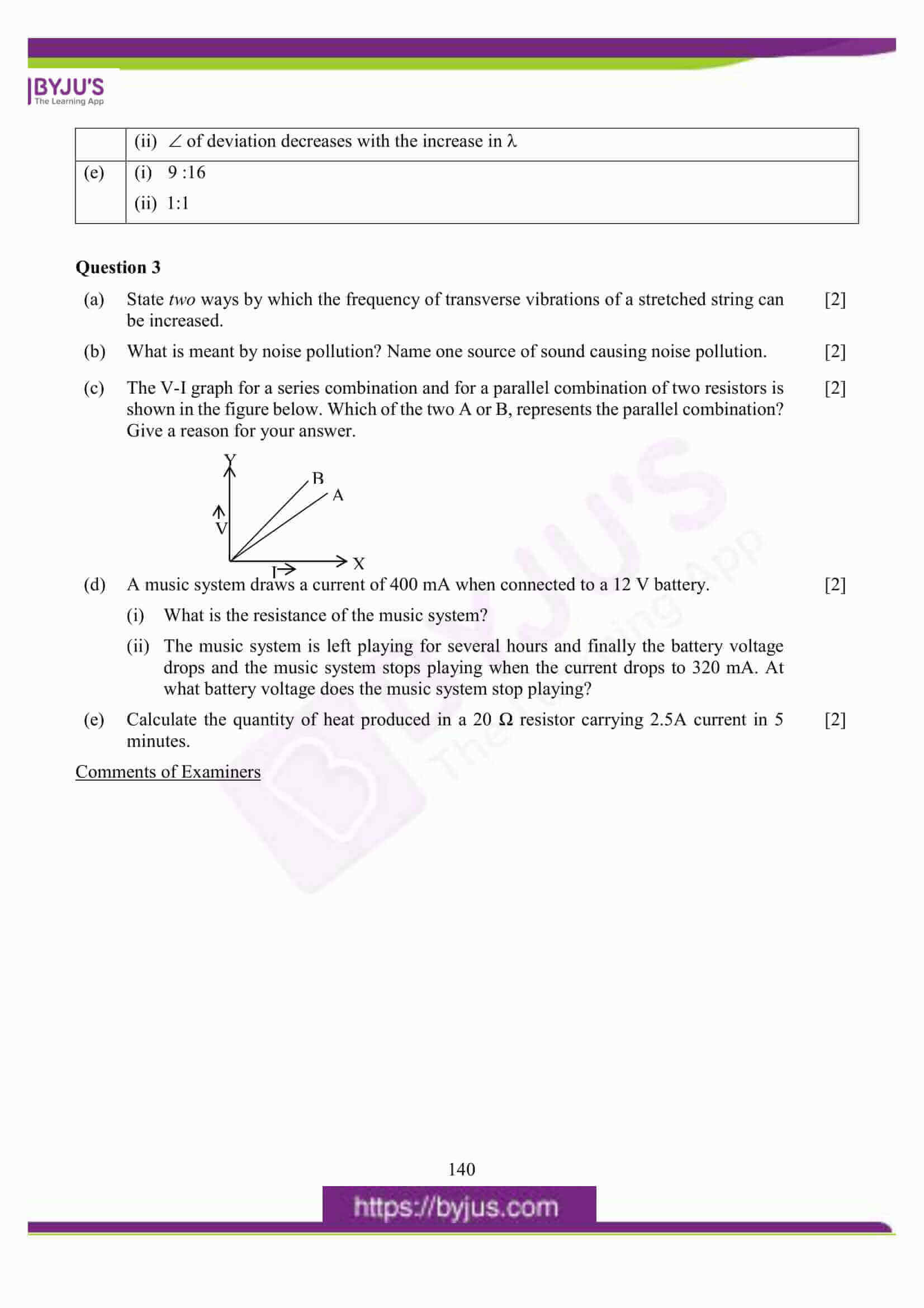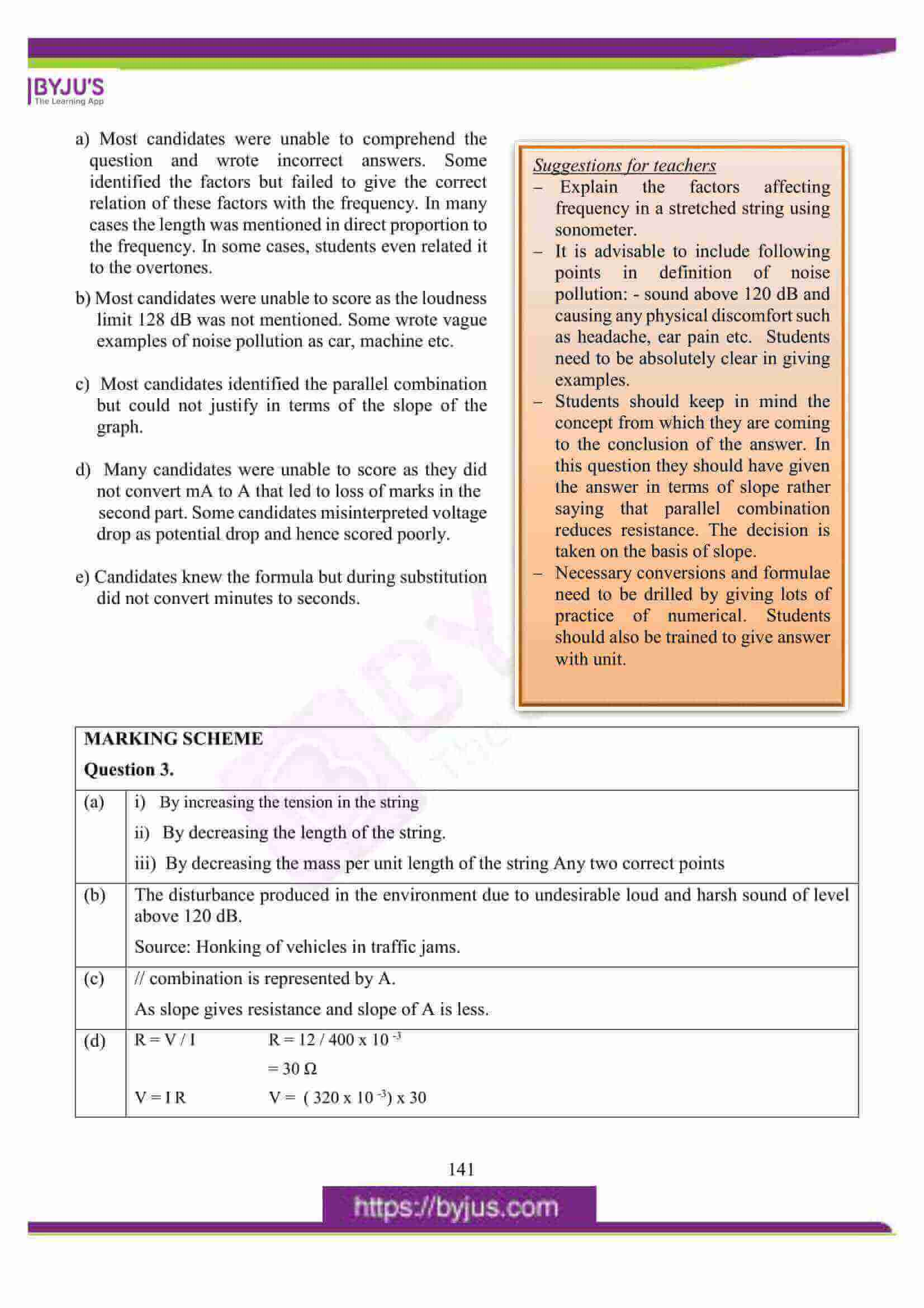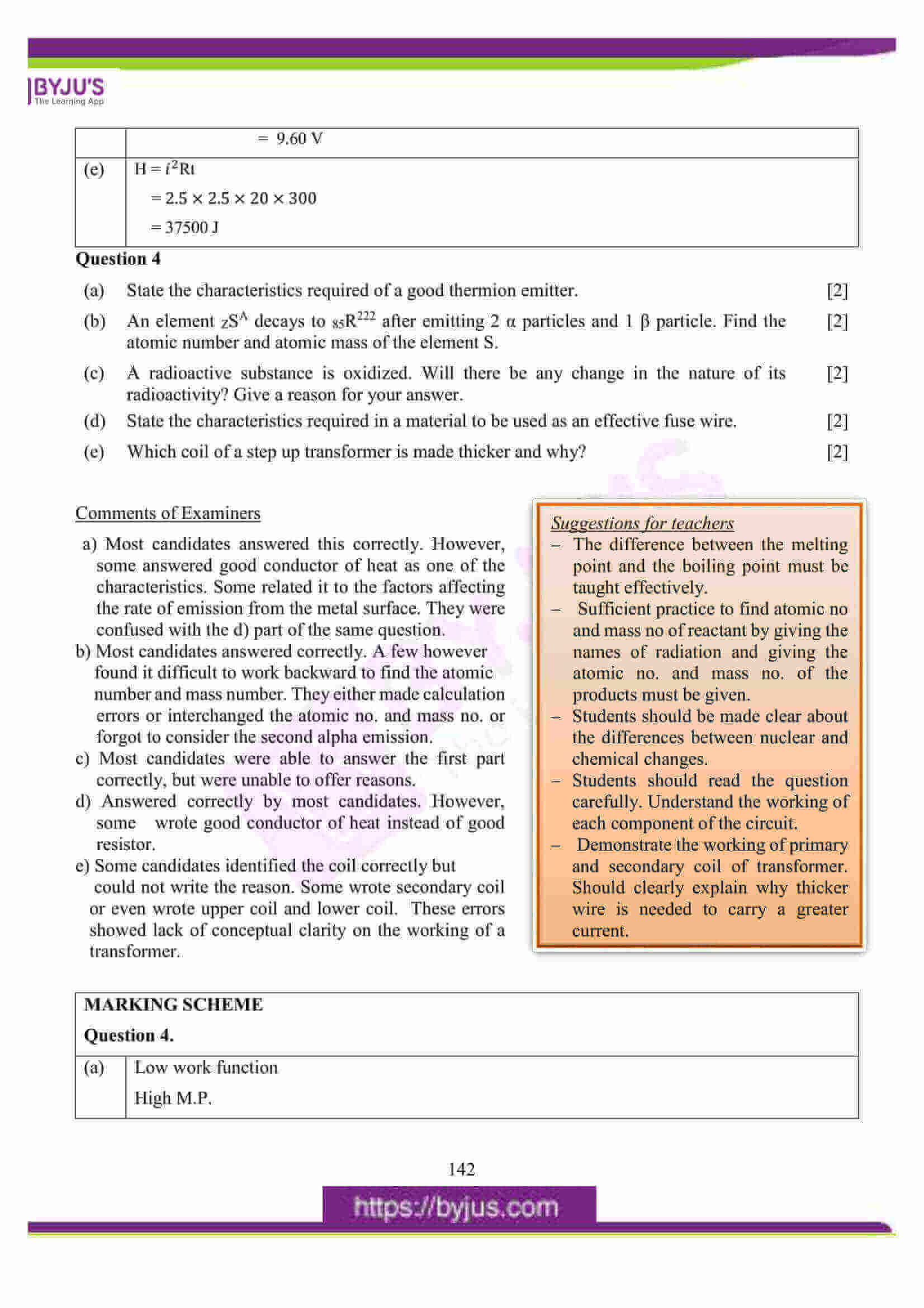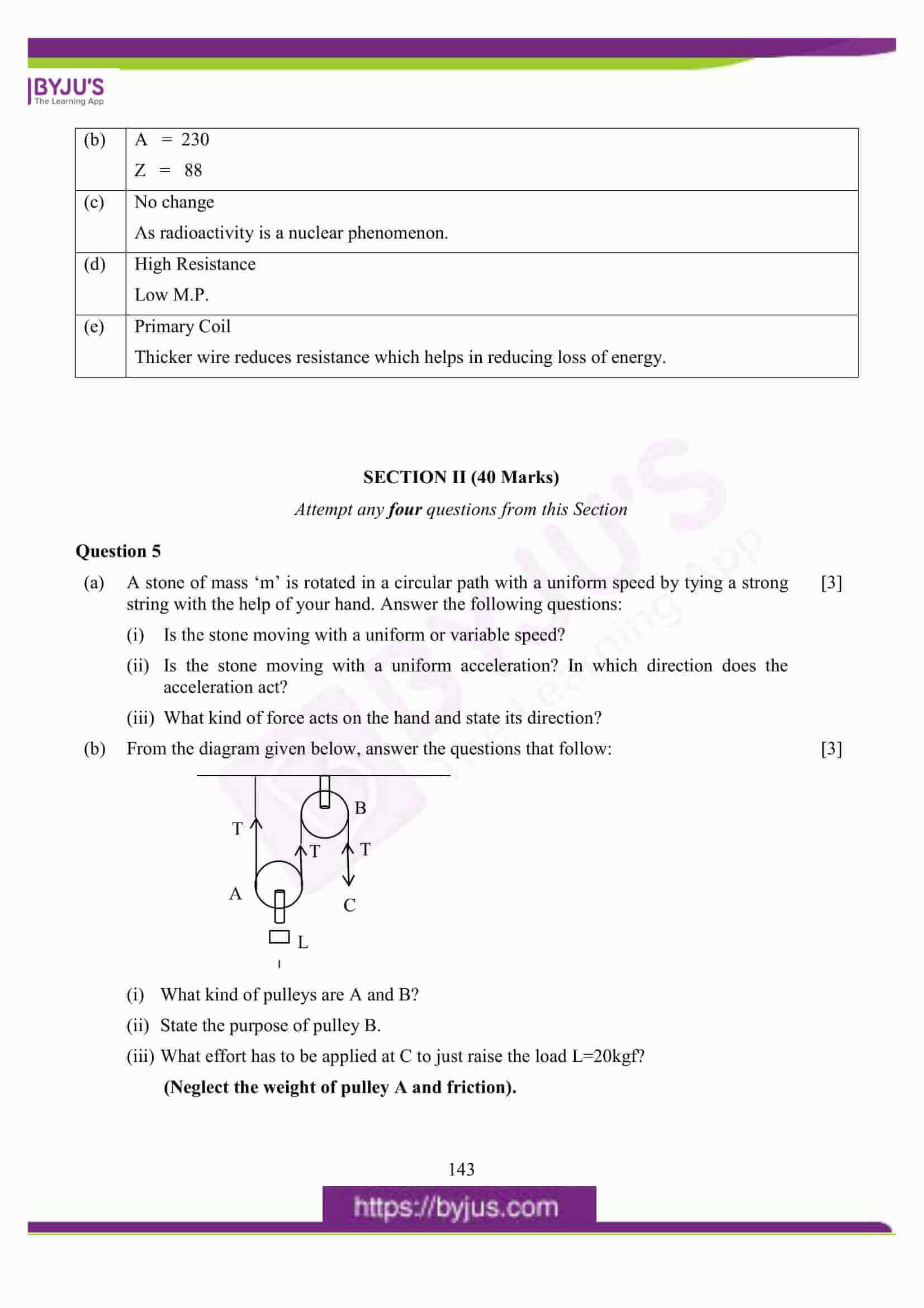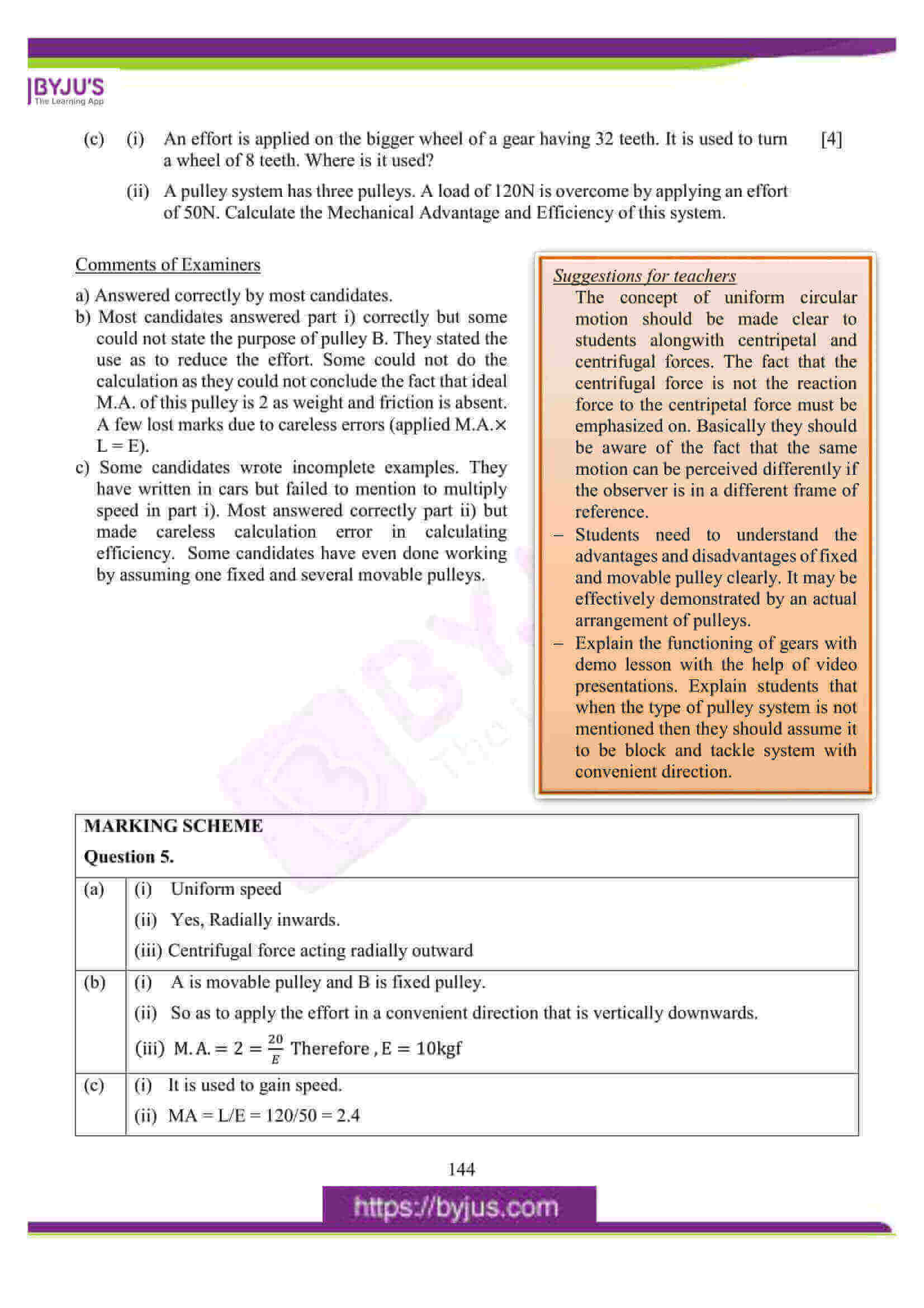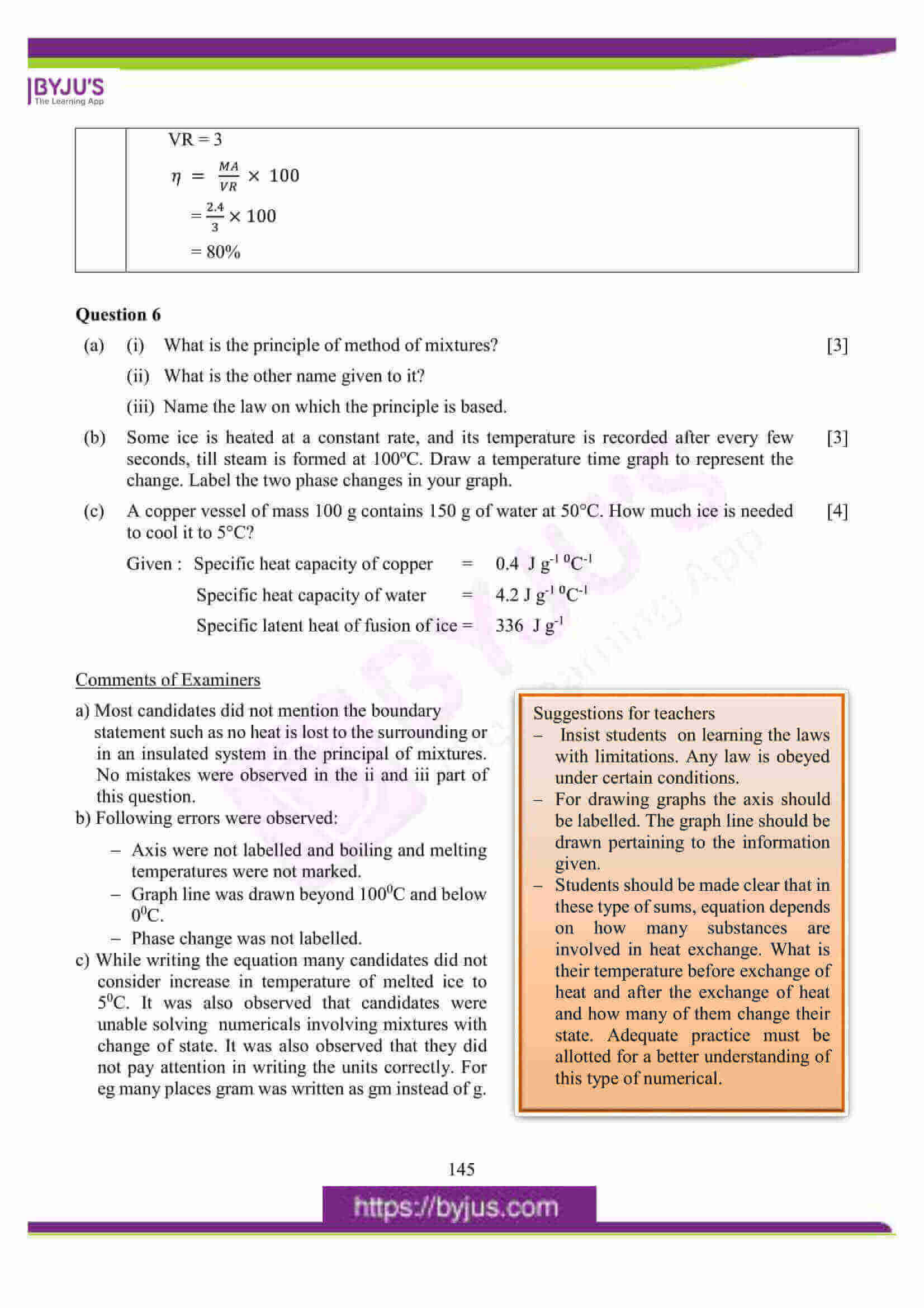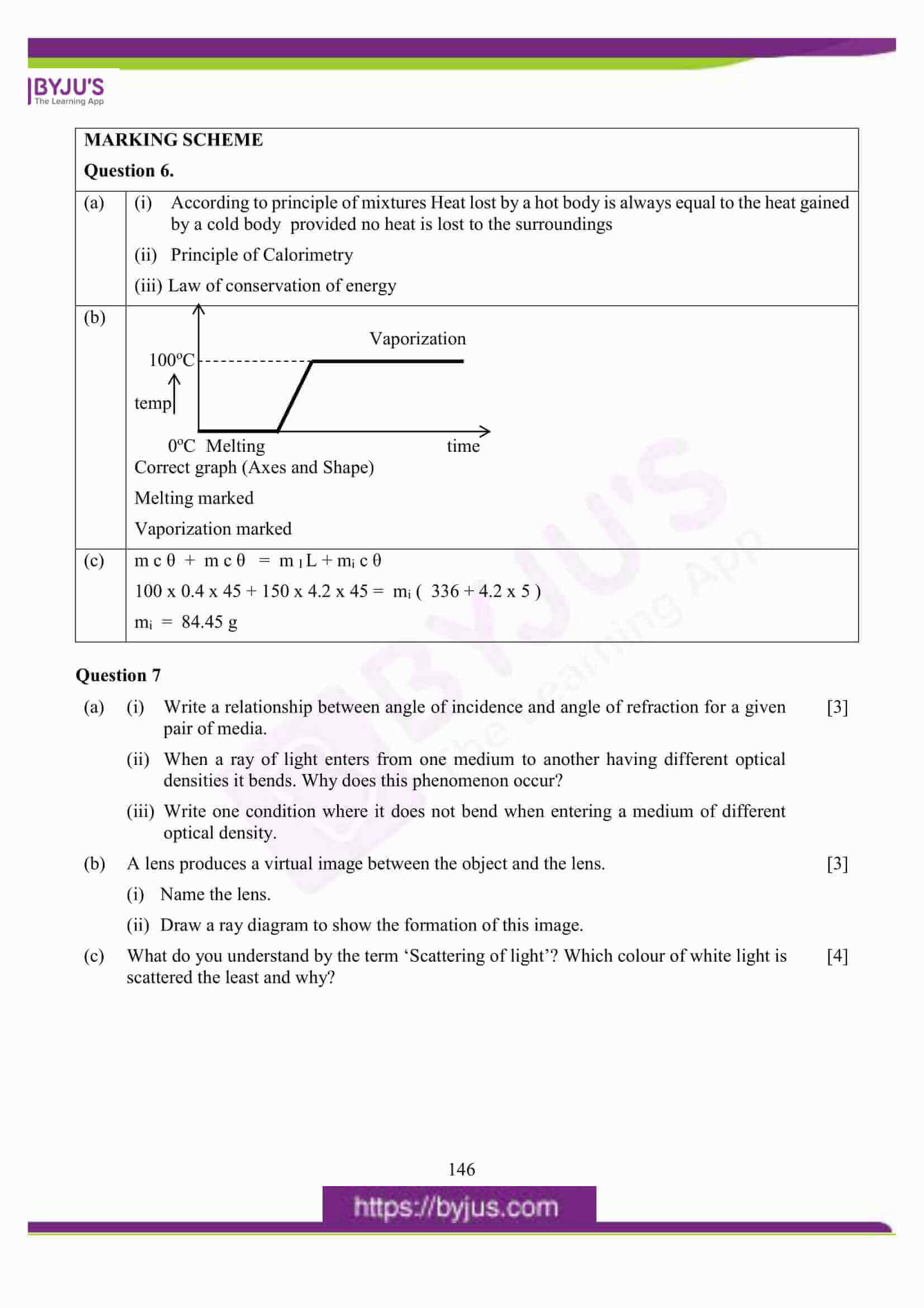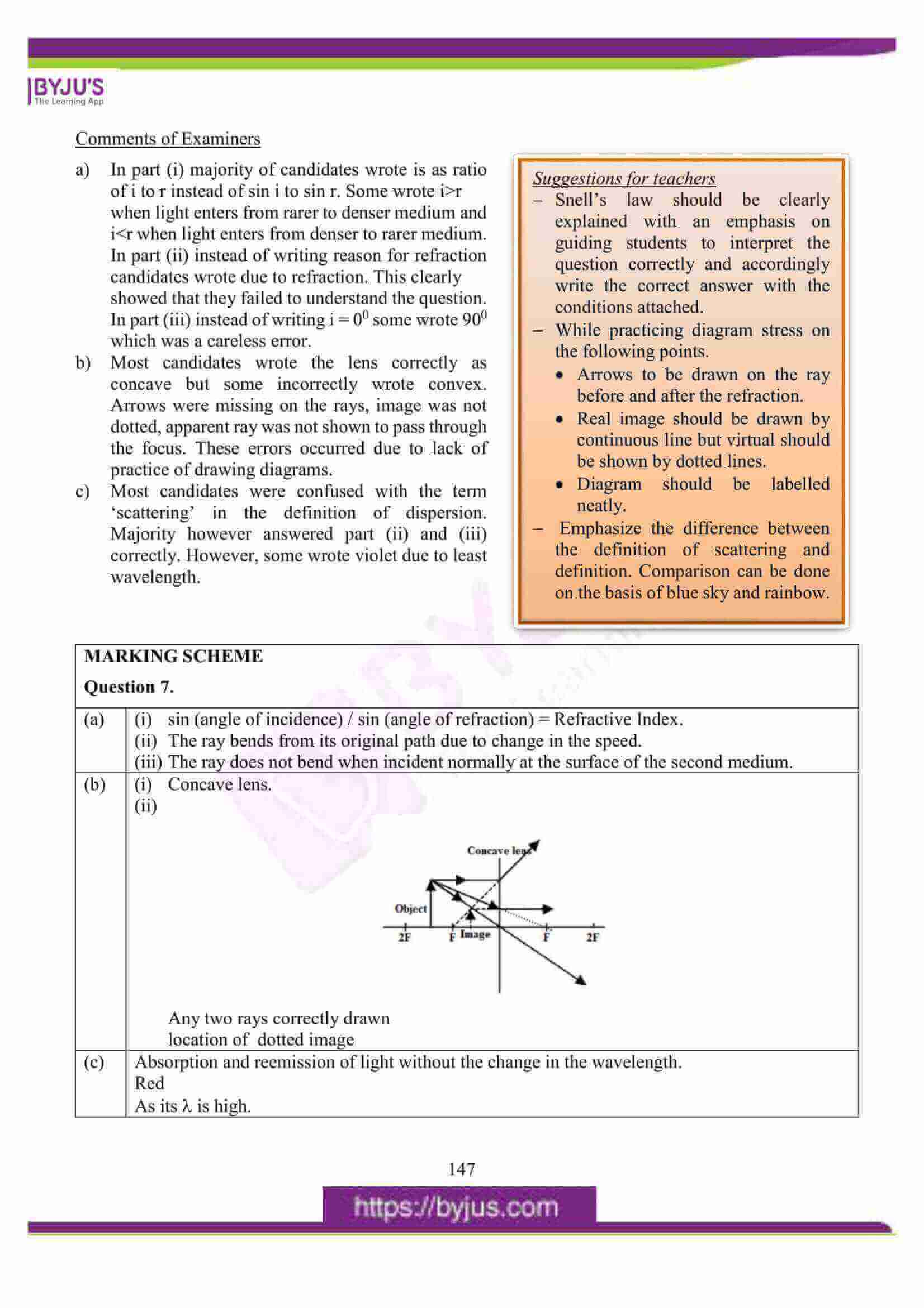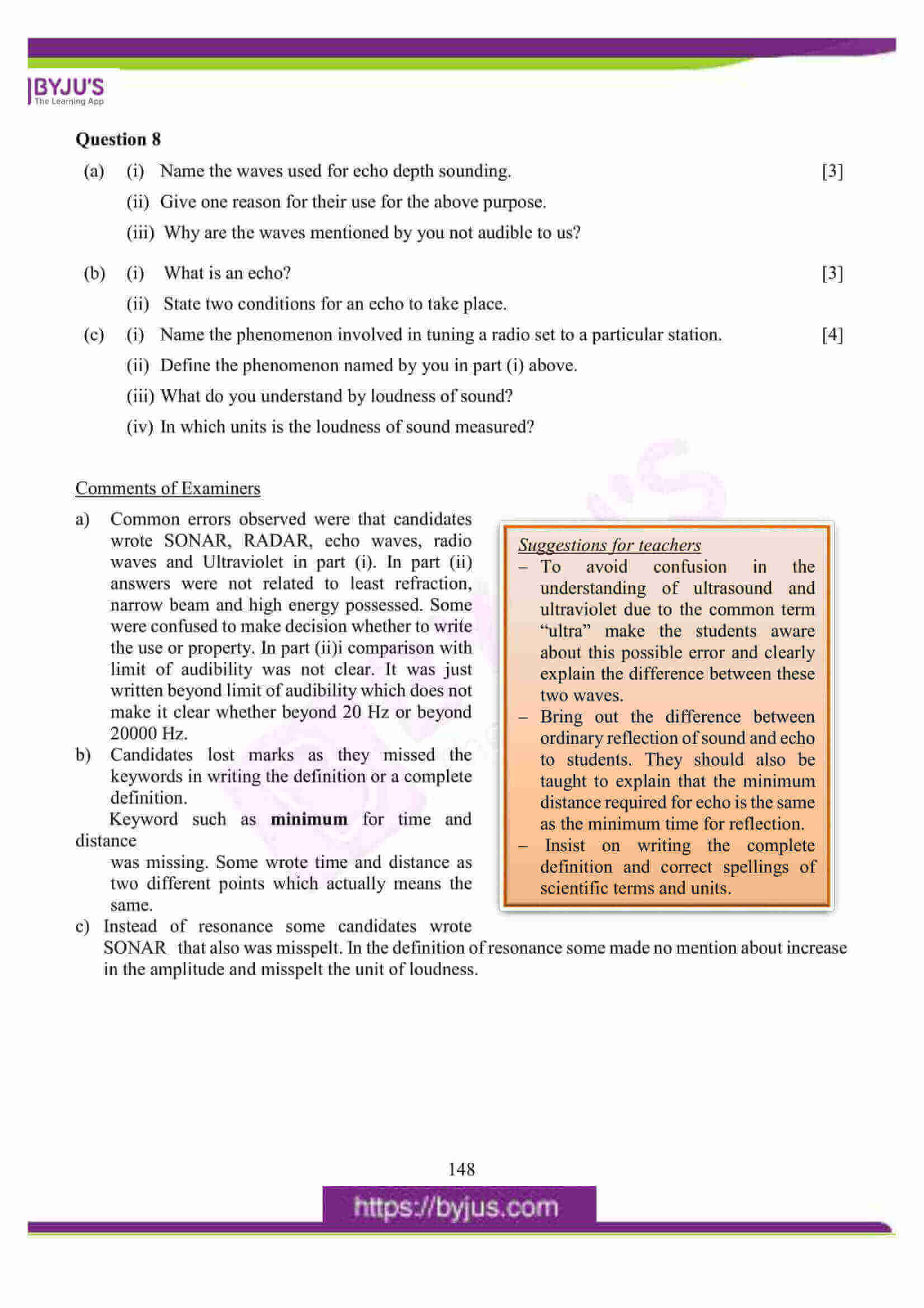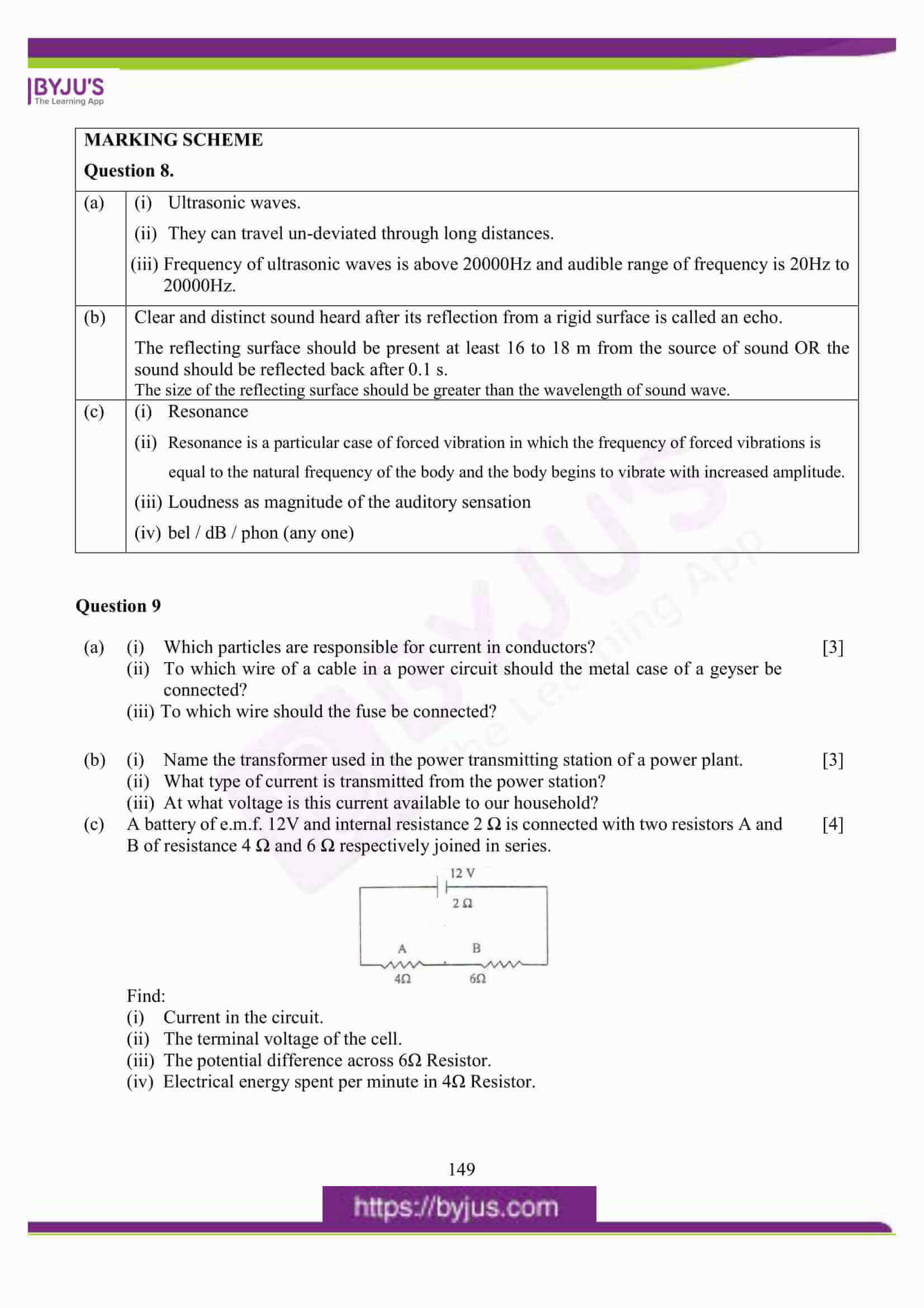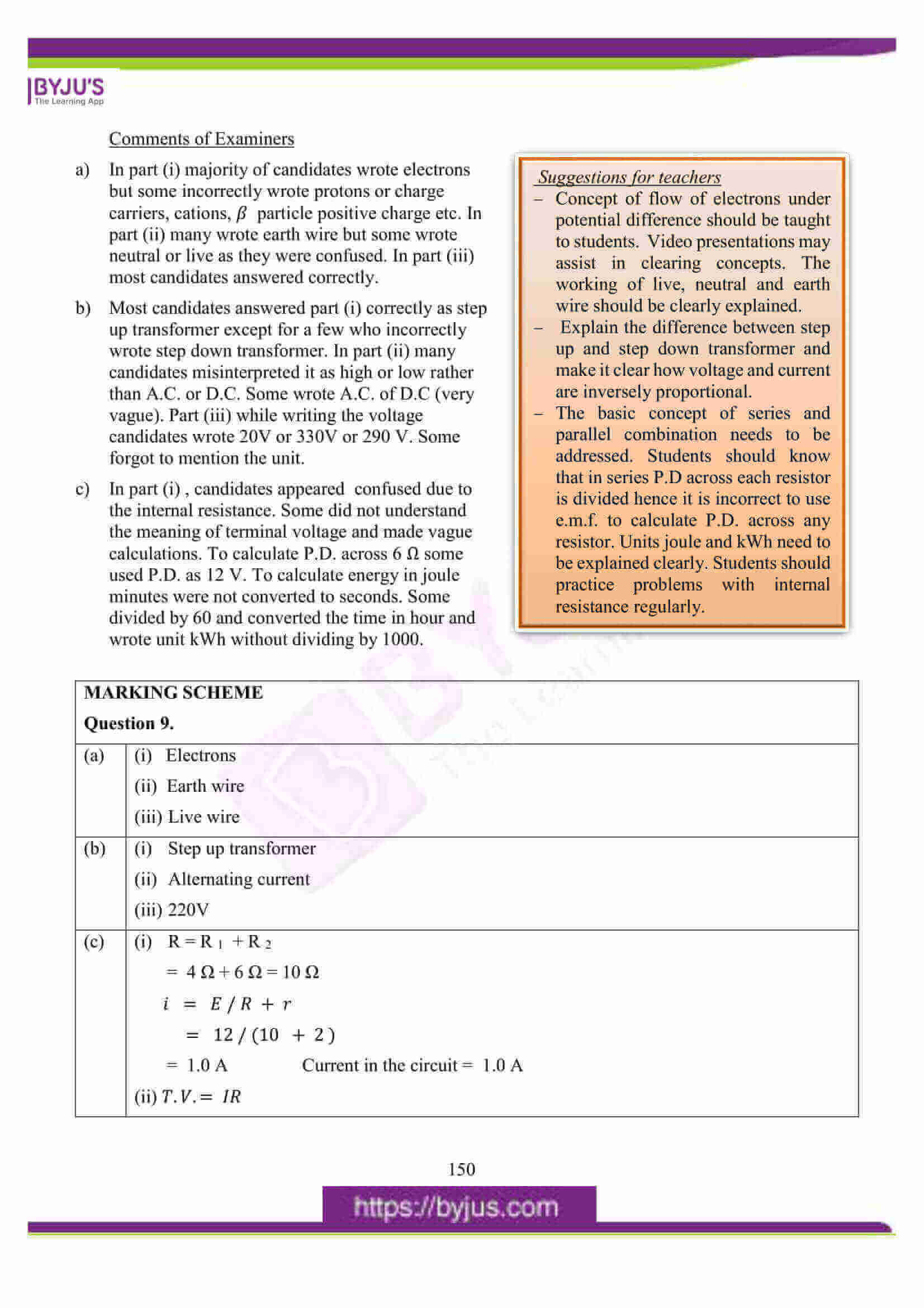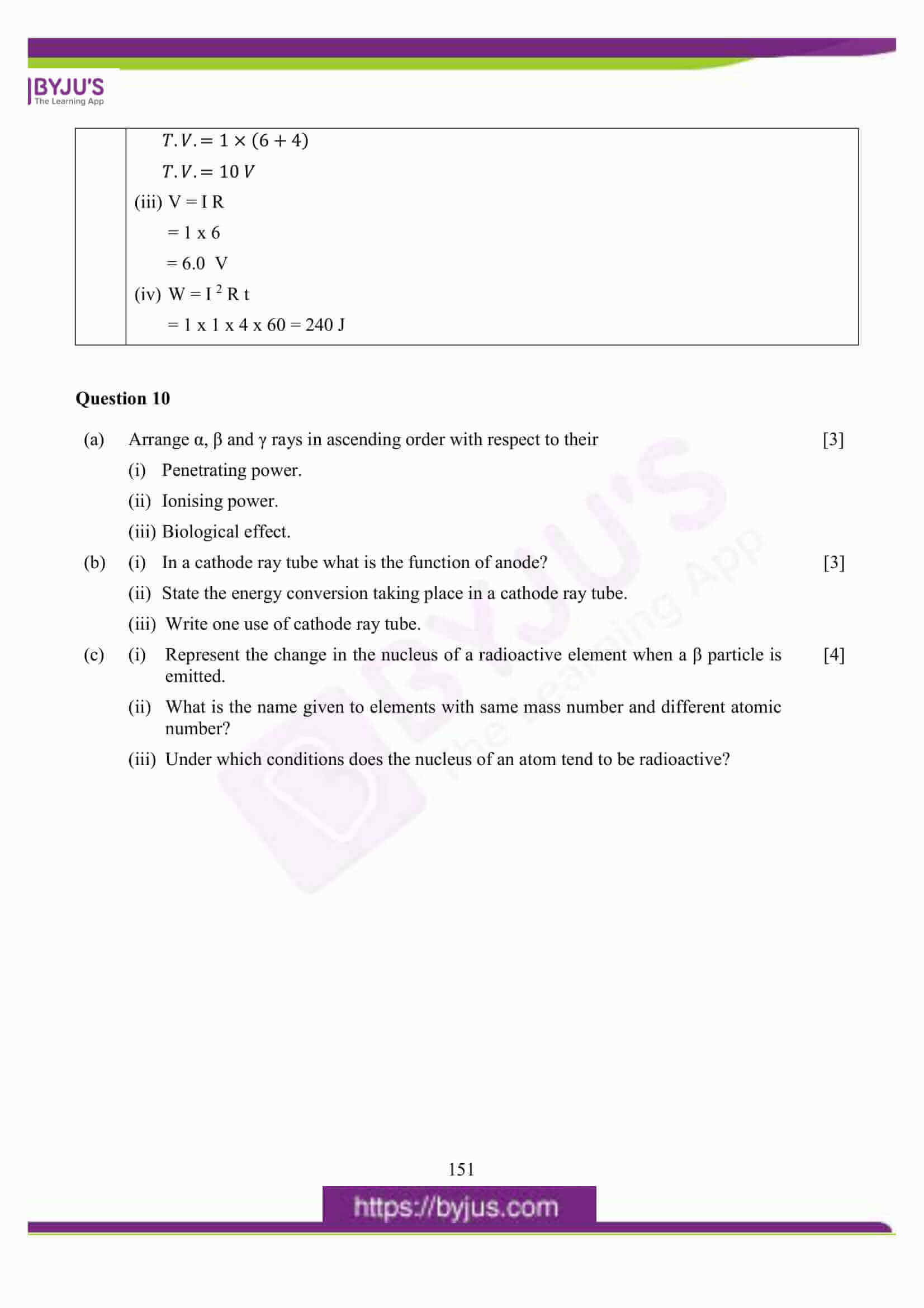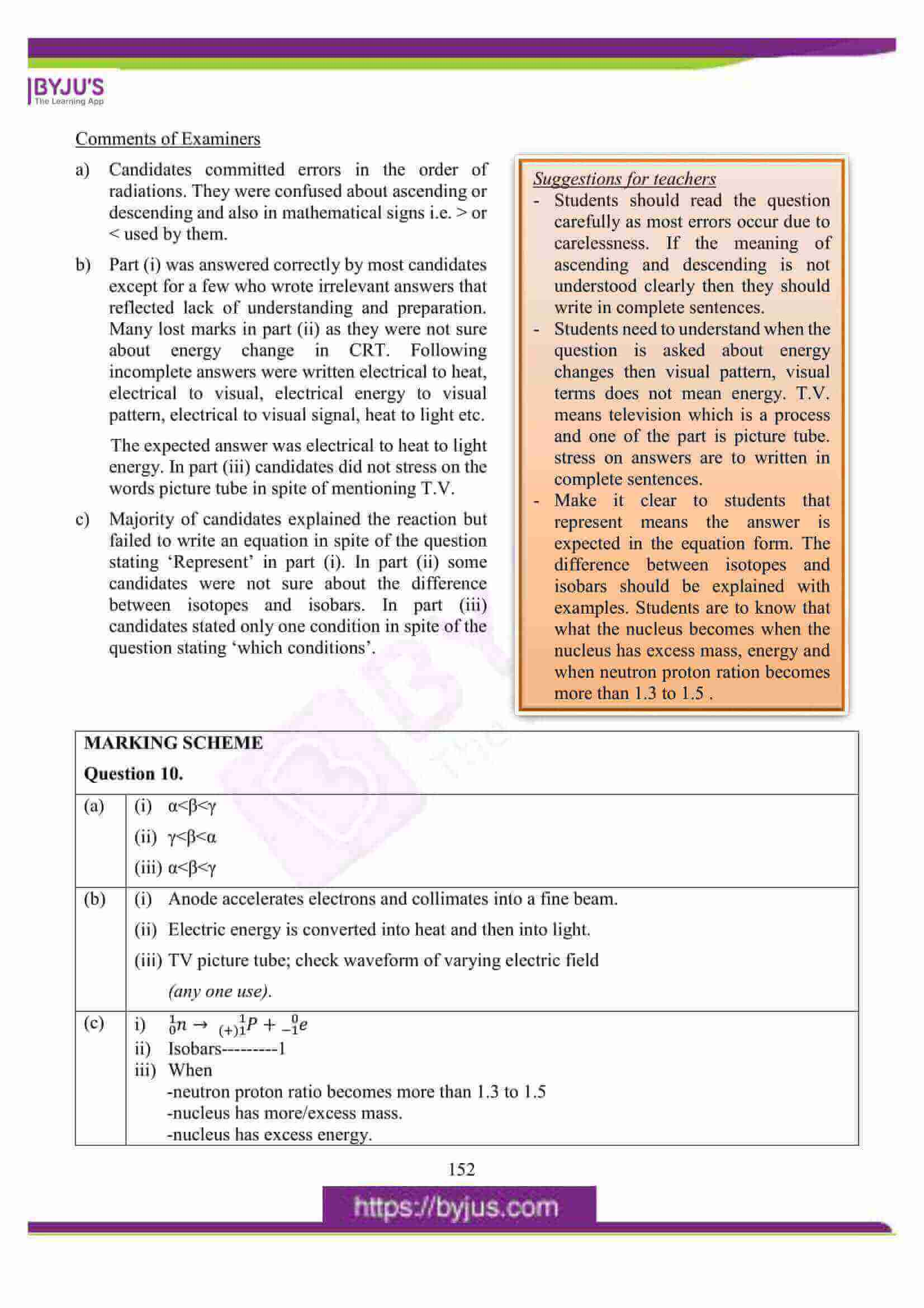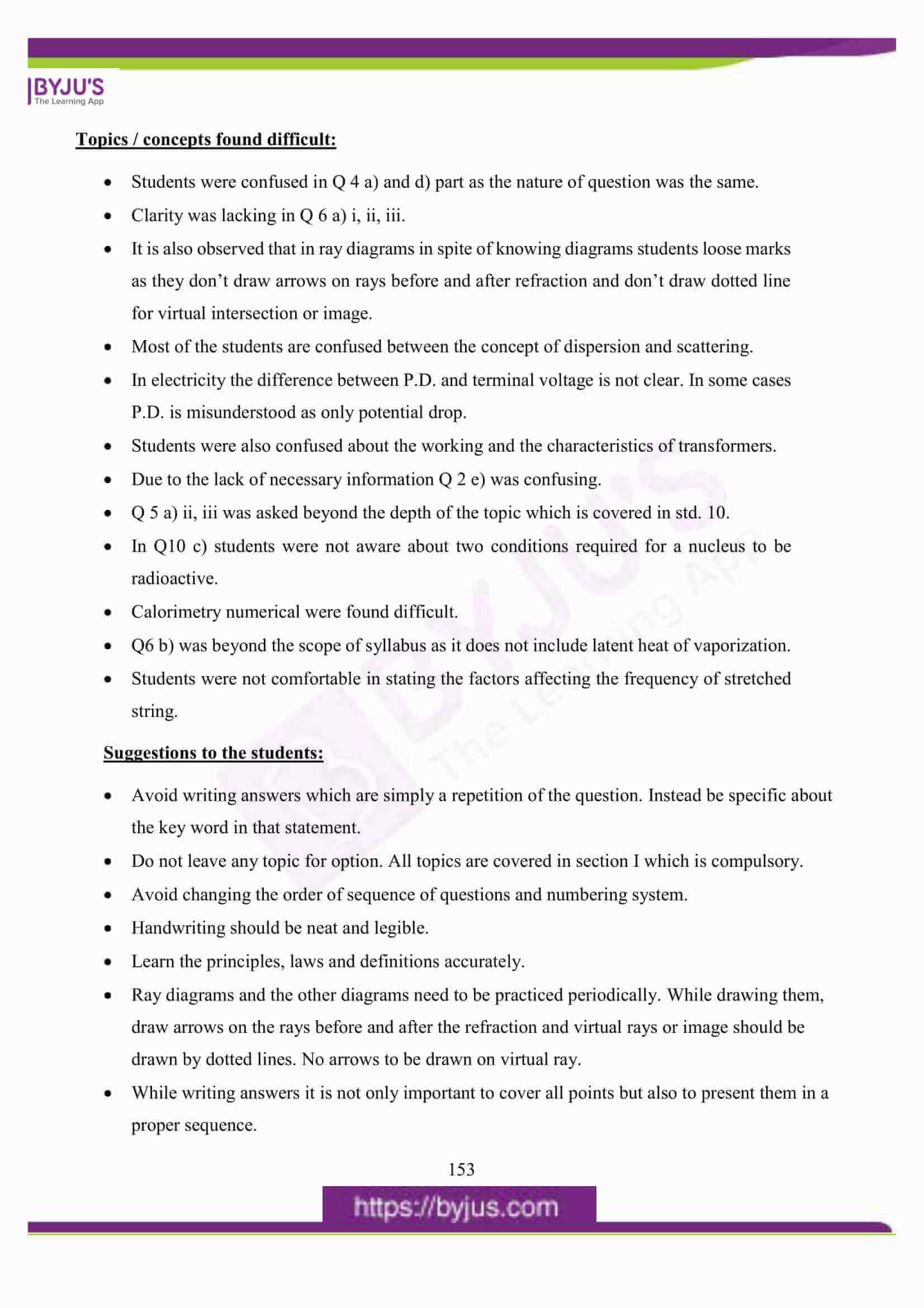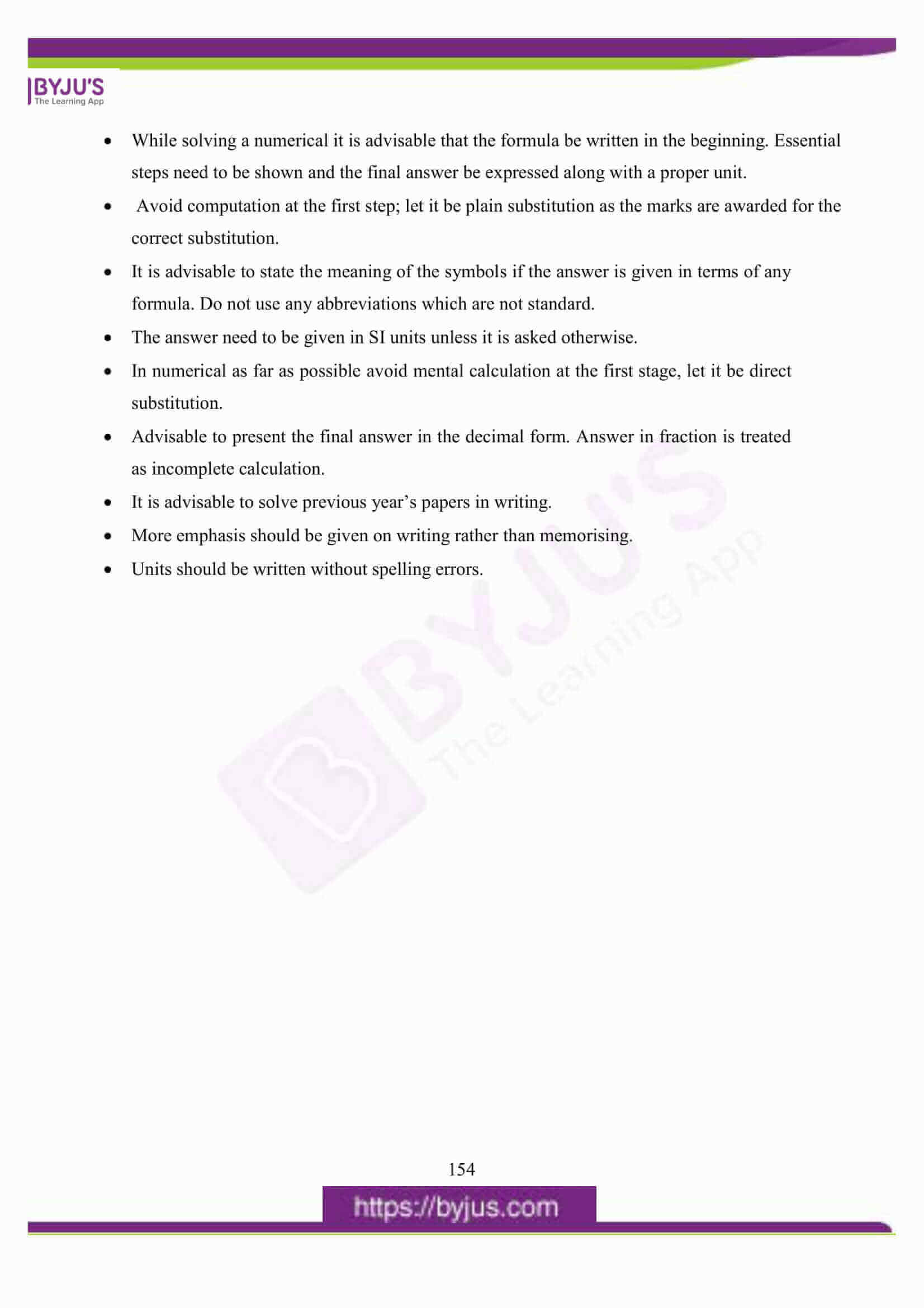## ICSE Class 10 Physics (Science Paper 1) Question Paper 2016 With Solution

### Question 1:

(a) (i) Give an example of a non-contact force which is always of attractive nature.

(ii) How does the magnitude of this non-contact force on the two bodies depend on the distance of separation between them?

(b) A boy weighing 40kgf climbs up a stair of 30 steps each 20cm high in 4 minute and a girl weighing 30kgf does the same in 3 minutes. Compare:-

(i) The work done by them.

(ii) The power developed by them.

(c) With reference to the terms Mechanical Advantage, Velocity Ratio and efficiency of a

machine, name and define the term that will not change for a machine of a given design.

(d) Calculate the mass of ice required to lower the temperature of 300g of water at 40°C to water at 0°C.

(Specific latent heat of ice=336 J/g, Specific heat capacity of water=4.2 J/goC)

(e) What do you understand by the following statements:

(i) The heat capacity of the body is 60 JK-1.

(ii) The specific heat capacity of lead is 130 Jkg-1K-1.

(a) (i) Gravitational force

(ii) Magnitude of non-contact forces on the two bodies is inversely proportional to the Square of the distance of separation between them.

(b) i) Work done by the boy = 40×10×6 = 2400J

Work done by the girl = 30×10×6 = 1800J

Therefore, Ratio = 2400:1800 = 4:3

ii) Power of boy = 2400/(4×60) = 10 watt

Power of girl = 1800/(3×60) = 10 watt

Therefore Ratio = 10:10 = 1:1

(c) (i) Velocity Ratio

(ii) Ratio of velocity of effort to the velocity of effort Or ratio of displacement of effort to the

displacement of load in the same time.

(d) 𝑚 × 336 = 300 × 4.2 × 40

m = (300 × 4.2 × 40) / 336 = 150 g

(e) (i) 60 J of heat energy is required to raise the temperature of a body by 1K.

(ii) Lead requires 130 J of heat energy to raise the temperature of 1 Kg by 1K.

### Question 2:

(a) State two factors upon which the heat absorbed by a body depends.

(b) A boy uses blue colour of light to find the refractive index of glass. He then repeats the experiment using the red colour of light. Will the refractive index be the same or different in the two cases? Give a reason to support your answer.

(c) Copy the diagram given below and complete the path of light ray till it emerges out of the prism. The critical angle of glass is 42°. In your diagram mark the angles wherever necessary.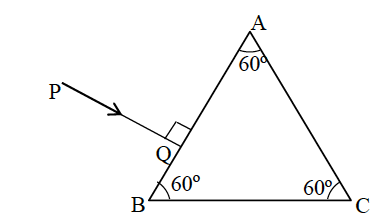(d) State the dependence of angle of deviation:

(i) On the refractive index of the material of the prism.

(ii) On the wavelength of light.

(e) The ratio of amplitude of two waves is 3 : 4. What is the ratio of their:

(i) loudness?

(ii) frequencies?

(a)

– Directly proportional to the mass.

– Directly proportional to change in temperature.

– Directly proportional to the specific heat capacity. Any two factors.

(b) Refractive index will be different.

a𝜇g= sin 𝑖 ⁄ sin 𝑟, different colours will have different angle of refraction even if the angle of incidence is the same therefore refractive index will be different. OR Speed of red colour and blue colour of light in air or vacuum is the same but in glass it is different. So the refractive index of glass will be different as aμg = Ca/Cb.

(c)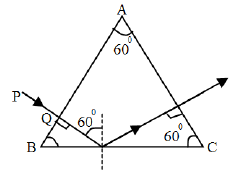(d) (i) of deviation decreases with the decrease in

(ii) of deviation decreases with the increase in λ

(e) (i) 9 :16

(ii) 1:1

### Question 3:

(a) State two ways by which the frequency of transverse vibrations of a stretched string can be increased.

(b) What is meant by noise pollution? Name one source of sound causing noise pollution.

(c) The V-I graph for a series combination and for a parallel combination of two resistors is shown in the figure below. Which of the two A or B, represents the parallel combination? Give a reason for your answer.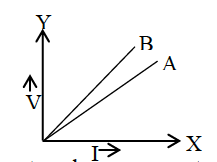(d) A music system draws a current of 400 mA when connected to a 12 V battery.

(i) What is the resistance of the music system?

(ii) The music system is left playing for several hours and finally the battery voltage drops and the music system stops playing when the current drops to 320 mA. At what battery voltage does the music system stop playing?

(e) Calculate the quantity of heat produced in a 20 Ω resistor carrying 2.5A current in 5 minutes.

(a) i) By increasing the tension in the string

ii) By decreasing the length of the string.

iii) By decreasing the mass per unit length of the string Any two correct points

(b) The disturbance produced in the environment due to undesirable loud and harsh sound of level above 120 dB.

Source: Honking of vehicles in traffic jams.

(c) // combination is represented by A.

As slope gives resistance and slope of A is less.

(d)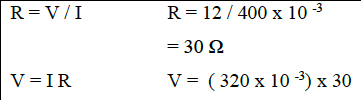= 9.60 V

(e) H = 𝑖2Rt

= 2.5 × 2.5 × 20 × 300

= 37500 J

### Question 4:

(a) State the characteristics required of a good thermion emitter.

(b) An element ZSA decays to 85R222 after emitting 2 α particles and 1 β particle. Find the atomic number and atomic mass of the element S.

(c) A radioactive substance is oxidized. Will there be any change in the nature of its radioactivity? Give a reason for your answer.

(d) State the characteristics required in a material to be used as an effective fuse wire.

(e) Which coil of a step up transformer is made thicker and why?

(a) Low work function

High M.P.

(b) A = 230

Z = 88

(c) No change

As radioactivity is a nuclear phenomenon.

(d) High Resistance

Low M.P.

(e) Primary Coil

Thicker wire reduces resistance which helps in reducing loss of energy.

### Question 5:

(a) A stone of mass ‘m’ is rotated in a circular path with a uniform speed by tying a strong string with the help of your hand. Answer the following questions:

(i) Is the stone moving with a uniform or variable speed?

(ii) Is the stone moving with a uniform acceleration? In which direction does the acceleration act?

(iii) What kind of force acts on the hand and state its direction?

(b) From the diagram given below, answer the questions that follow: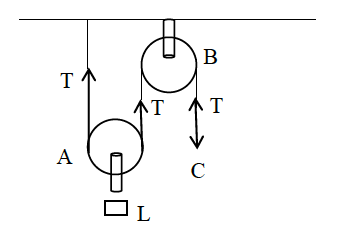(i) What kind of pulleys are A and B?

(ii) State the purpose of pulley B.

(iii) What effort has to be applied at C to just raise the load L=20kgf? (Neglect the weight of pulley A and friction).

(c) (i) An effort is applied on the bigger wheel of a gear having 32 teeth. It is used to turn a wheel of 8 teeth. Where is it used?

(ii) A pulley system has three pulleys. A load of 120N is overcome by applying an effort of 50N. Calculate the Mechanical Advantage and Efficiency of this system.

(a) (i) Uniform speed

(iii) Centrifugal force acting radially outward

(b) (i) A is movable pulley and B is fixed pulley.

(ii) So as to apply the effort in a convenient direction that is vertically downwards.

(iii) M. A. = 2 = 20/𝐸

Therefore , E = 10kgf

(c) (i) It is used to gain speed.

(ii) MA = L/E = 120/50 = 2.4

VR = 3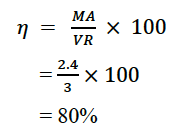### Question 6:

(a) (i) What is the principle of method of mixtures?

(ii) What is the other name given to it?

(iii) Name the law on which the principle is based.

(b) Some ice is heated at a constant rate, and its temperature is recorded after every few seconds, till steam is formed at 100oC. Draw a temperature time graph to represent the change. Label the two phase changes in your graph.

(c) A copper vessel of mass 100 g contains 150 g of water at 50°C. How much ice is needed

to cool it to 5°C?

Given : Specific heat capacity of copper = 0.4 J g-1 ⁰C-1

Specific heat capacity of water = 4.2 J g-1⁰C-1

Specific latent heat of fusion of ice = 336 J g-1

(a) (i) According to principle of mixtures Heat lost by a hot body is always equal to the heat gained by a cold body provided no heat is lost to the surroundings

(ii) Principle of Calorimetry

(iii) Law of conservation of energy

(b)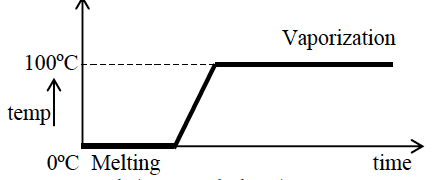Correct graph (Axes and Shape)

Melting marked

Vaporization marked

(c) m c θ + m c θ = m I L + mi c θ

100 x 0.4 x 45 + 150 x 4.2 x 45 = mi ( 336 + 4.2 x 5 )

mi = 84.45 g

### Question 7:

(a) (i) Write a relationship between angle of incidence and angle of refraction for a given pair of media.

(ii) When a ray of light enters from one medium to another having different optical densities it bends. Why does this phenomenon occur?

(iii) Write one condition where it does not bend when entering a medium of different optical density.

(b) A lens produces a virtual image between the object and the lens.

(i) Name the lens.

(ii) Draw a ray diagram to show the formation of this image.

(c) What do you understand by the term ‘Scattering of light’? Which colour of white light is scattered the least and why?

(a) (i) sin (angle of incidence) / sin (angle of refraction) = Refractive Index.

(ii) The ray bends from its original path due to change in the speed.

(iii) The ray does not bend when incident normally at the surface of the second medium.

(b) (i) Concave lens.

(ii)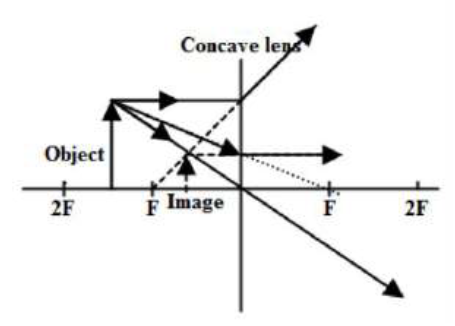Any two rays correctly drawn

location of dotted image

(c) Absorption and reemission of light without the change in the wavelength. Red

As its λ is high.

### Question 8:

(a) (i) Name the waves used for echo depth sounding.

(ii) Give one reason for their use for the above purpose.

(iii) Why are the waves mentioned by you not audible to us?

(b) (i) What is an echo?

(ii) State two conditions for an echo to take place.

(c) (i) Name the phenomenon involved in tuning a radio set to a particular station.

(ii) Define the phenomenon named by you in part (i) above.

(iii) What do you understand by loudness of sound?

(iv) In which units is the loudness of sound measured?

(a) (i) Ultrasonic waves.

(ii) They can travel un-deviated through long distances.

(iii) Frequency of ultrasonic waves is above 20000Hz and audible range of frequency is 20Hz to 20000Hz.

(b) Clear and distinct sound heard after its reflection from a rigid surface is called an echo.

The reflecting surface should be present at least 16 to 18 m from the source of sound OR the

sound should be reflected back after 0.1s.

The size of the reflecting surface should be greater than the wavelength of sound wave.

(c) (i) Resonance

(ii) Resonance is a particular case of forced vibration in which the frequency of forced vibrations is equal to the natural frequency of the body and the body begins to vibrate with increased amplitude.

(iii) Loudness as magnitude of the auditory sensation

(iv) bel / dB / phon (any one)

### Question 9:

(a) (i) Which particles are responsible for current in conductors?

(ii) To which wire of a cable in a power circuit should the metal case of a geyser be connected?

(iii) To which wire should the fuse be connected?

(b) (i) Name the transformer used in the power transmitting station of a power plant.

(ii) What type of current is transmitted from the power station?

(iii) At what voltage is this current available to our household?

(c) A battery of e.m.f. 12V and internal resistance 2 Ω is connected with two resistors A and B of resistance 4 Ω and 6 Ω respectively joined in series.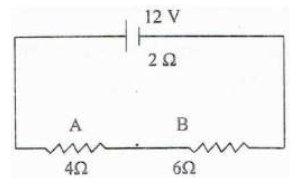Find:

(i) Current in the circuit.

(ii) The terminal voltage of the cell.

(iii) The potential difference across 6Ω Resistor.

(iv) Electrical energy spent per minute in 4Ω Resistor.

(a) (i) Electrons

(ii) Earth wire

(iii) Live wire

(b) (i) Step up transformer

(ii) Alternating current

(iii) 220V

(c) (i) R = R1 + R2

= 4Ω + 6Ω = 10Ω

𝑖 = 𝐸 / 𝑅 + 𝑟

= 12 / (10 + 2 )

= 1.0 A

Current in the circuit = 1.0 A

(ii) 𝑇. 𝑉. = 𝐼𝑅

𝑇. 𝑉. = 1 × (6 + 4)

𝑇. 𝑉. = 10 𝑉

(iii) V = I R

= 1 x 6

= 6.0 V

(iv) W = I2 R t

= 1 x 1 x 4 x 60 = 240 J

### Question 10:

(a) Arrange α, β and γ rays in ascending order with respect to their

(i) Penetrating power.

(ii) Ionising power.

(iii) Biological effect.

(b) (i) In a cathode ray tube what is the function of anode?

(ii) State the energy conversion taking place in a cathode ray tube.

(iii) Write one use of cathode ray tube.

(c) (i) Represent the change in the nucleus of a radioactive element when a β particle is emitted.

(ii) What is the name given to elements with the same mass number and different atomic number?

(iii) Under which conditions does the nucleus of an atom tend to be radioactive?

(a) (i) α<β<γ

(ii) γ<β<α

(iii) α<β<γ

(b) (i) Anode accelerates electrons and collimates into a fine beam.

(ii) Electric energy is converted into heat and then into light.

(iii) TV picture tube; check waveform of varying electric fields (any one use).

(c) i)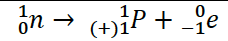ii) Isobars———1

iii) When

– neutron proton ratio becomes more than 1.3 to 1.5

– nucleus has more/excess mass.

– nucleus has excess energy.

We hope ICSE Class 10 Physics Question Paper Solution 2016 must have provided an insight into the 2016 Question paper and their solutions. Going through this paper solution will surely help students in their exam preparation. Students can also find the answers of other papers of ICSE Class 10 Previous Years Questions by clicking here. Happy Learning and stay tuned to BYJU’S for the latest update on ICSE/CBSE/State Boards/Competitive exams. Also, don’t forget to download the BYJU’S App.